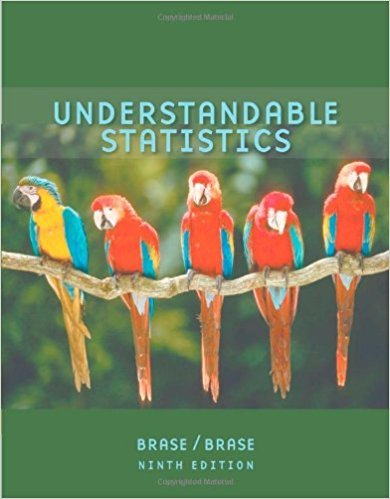×
×

# Civil Engineering: Soil Profiles Sand and clay studiesISBN: 9780618949922 213

## Solution for problem 9 Chapter 12.4

Understandable Statistics | 9th Edition

• Textbook Solutions
• 2901 Step-by-step solutions solved by professors and subject experts
• Get 24/7 help from StudySoup virtual teaching assistantsUnderstandable Statistics | 9th Edition

4 5 1 355 Reviews
17
4
Problem 9

Civil Engineering: Soil Profiles Sand and clay studies were conducted at the West Side Field Station of the University of California. (Reference: Professor D. R. Nielsen, University of California, Davis.) Twelve consecutive depths, each about 15 cm deep, were studied and the following percentages of sand in the soil were recorded. 19.0 27.0 30.0 24.3 33.2 27.5 24.2 18.0 16.2 8.3 1.0 0.0 (i) Convert this sequence of numbers to a sequence of symbols A and B, where A indicates a value above the median and B a value below the median. (ii) Test the sequence for randomness about the median. Use a 0.05.

Step-by-Step Solution:
Step 1 of 3

Chapter 1 Section 1 Variable-a characteristic/attribute that can assume different values Data- values that the variables can assume Population- all subjects undergoing a study Parameter- measure obtained from the population data  Uses Greek Letters to categorize Sample- a group selected from the population, to make the study more concentrated...

Step 2 of 3

Step 3 of 3

##### ISBN: 9780618949922

This textbook survival guide was created for the textbook: Understandable Statistics, edition: 9. This full solution covers the following key subjects: . This expansive textbook survival guide covers 57 chapters, and 994 solutions. The answer to “Civil Engineering: Soil Profiles Sand and clay studies were conducted at the West Side Field Station of the University of California. (Reference: Professor D. R. Nielsen, University of California, Davis.) Twelve consecutive depths, each about 15 cm deep, were studied and the following percentages of sand in the soil were recorded. 19.0 27.0 30.0 24.3 33.2 27.5 24.2 18.0 16.2 8.3 1.0 0.0 (i) Convert this sequence of numbers to a sequence of symbols A and B, where A indicates a value above the median and B a value below the median. (ii) Test the sequence for randomness about the median. Use a 0.05.” is broken down into a number of easy to follow steps, and 104 words. The full step-by-step solution to problem: 9 from chapter: 12.4 was answered by , our top Statistics solution expert on 01/04/18, 09:09PM. Since the solution to 9 from 12.4 chapter was answered, more than 222 students have viewed the full step-by-step answer. Understandable Statistics was written by and is associated to the ISBN: 9780618949922.

Unlock Textbook Solution